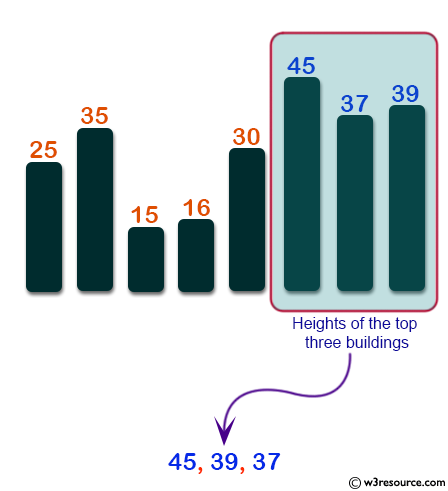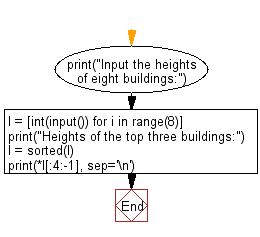﻿ Python: Find heights of the top three building in descending order from eight given buildings - w3resource# Python: Find heights of the top three building in descending order from eight given buildings

## Python Basic - 1: Exercise-32 with Solution

Write a python program to find heights of the top three building in descending order from eight given buildings.

Input:
0 ≤ height of building (integer) ≤ 10,000

Pictorial Presentation:Sample Solution:

Python Code:

``````print("Input the heights of eight buildings:")
l = [int(input()) for i in range(8)]
print("Heights of the top three buildings:")
l = sorted(l)
print(*l[:4:-1], sep='\n')
``````

Sample Output:

```Input the heights of eight buildings:
25
35
15
16
30
45
37
39
Heights of the top three buildings:
45
39
37
```

Flowchart:Python Code Editor:

Have another way to solve this solution? Contribute your code (and comments) through Disqus.

What is the difficulty level of this exercise?

Test your Programming skills with w3resource's quiz.

﻿

## Python: Tips of the Day

What is the difference between Python's list methods append and extend?

append: Appends object at the end.

```x = [1, 2, 3]
x.append([4, 5])
print (x)
```

Output:

```[1, 2, 3, [4, 5]]
```

extend: Extends list by appending elements from the iterable.

```x = [1, 2, 3]
x.extend([4, 5])
print (x)
```

Output:

```[1, 2, 3, 4, 5]
```

Ref: https://bit.ly/2AZ6ZFq